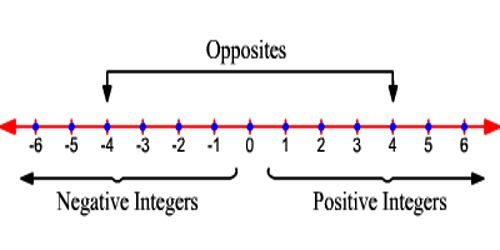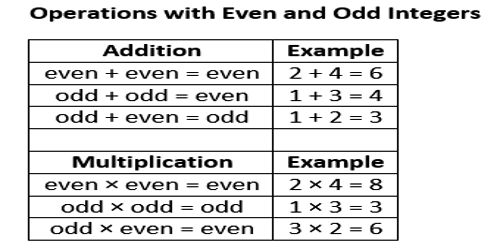Integers are rational numbers with a denominator of 1.

An integer is a whole number (not a fractional number) that can be positive, negative, or zero.

Examples of integers are: -5, 1, 5, 8, 97, and 3,043.

Examples of numbers that are not integers are: -1.43, 1 3/4, 3.14, .09, and 5,643.1.

A fraction is also a part of something (for example: 1/4 of a shape, 1/7 of a quantity) so is not an integer.RULE FOR ADDITION

The rule for adding integers states that when the signs are the same, you add (find the sum) of the “raw numbers” (absolute values) and use the same sign for your answer. If the signs are different, you find the difference, which is the answer in subtraction. and use the sign of the one which has the most (the bigger absolute value.)

Subtraction

You have several options how to present subtraction of integers. Personally, when subtracting a positive integer, I think in terms of jumps on the number line, and when subtracting a negative integer (“the double negative”), I change those to additions.

Number line. Here, 2 − 5 would mean that you start at 2 and move 5 units to the left, ending at −3. This is identical to interpreting the addition 2 + (−5) on the number line.

Similarly, −4 − 3 would mean that you start at −4 and move 3 units to the left, ending at −7. This is identical to interpreting the addition −4 + (−3) on the number line.

Subtracting a negative integer using number line movements is a bit trickier. Problem such as −4 − (−8) would mean that you start at −4, you get ready to move 8 units to the left (the “minus sign”), but the second minus sign reverses your direction, and you go 8 units to the right instead, ending at 4.

To add a positive and a negative integer (or a negative and a positive integer), follow these steps:

1. Find the absolute value of each integer.
2. Subtract the smaller number from the larger number you get in Step 1.
3. The result from Step 2 takes the sign of the integer with the greater absolute value.Some key points of Integers

• The number line goes on forever in both directions. This is indicated by the arrows.
• Whole numbers greater than zero are called positive integers. These numbers are to the right of zero on the number line.
• Whole numbers less than zero are called negative integers. These numbers are to the left of zero on the number line.
• The integer zero is neutral. It is neither positive nor negative.
• The sign of an integer is either positive (+) or negative (-), except zero, which has no sign.

Example: (1) The number of coins someone adds to or removes from a jar. If you add three coins to a jar, that would be represented by a positive value: +3. Removing three coins from a jar would be represented by a negative value: -3. Since you can only add or remove an entire coin from a jar (not half of or a third of a coin), integers would always represent the number of coins added or taken.

(2) Altitude, rounded to the nearest foot, is another example of an integer. Denver is at an altitude of 5,183 ft. Parts of New Orleans are at an altitude of -3 ft.

Information Sources: